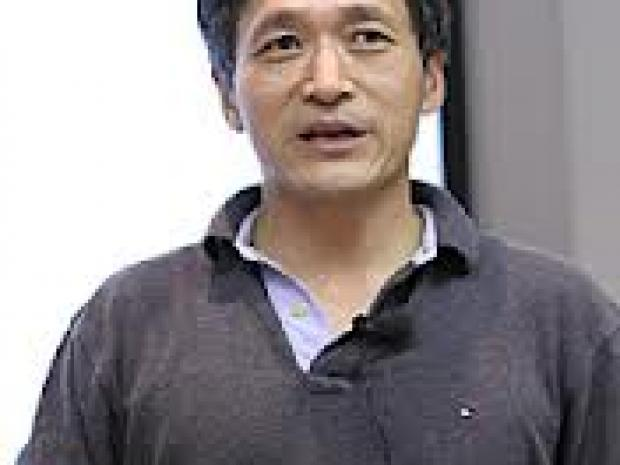Events

# On a Thousand Year Old Puzzle about Triangles- A Talk by Shou-Wu Zhang

Lecture / Panel

For NYU CommunityOn a Thousand Year Old Puzzle about Triangles
Do you know the area of a triangle?
Do you know how to construct all right triangles with rational sides?
If you know both, then try to solve a problem raised by Arabs over 1040
years ago:

Find a method to determine whether a positive integer n is the
area of a rational right triangle.

Such a number is called a “congruent number”.
Is 1 a congruent number? What about 5 or 7?

After 1000 years, we still do not know how to solve this problem. But it
follows from another unsolved problem, ne which has a prize of \$1,000,000.

——-Shou-Wu Zhang, Princeton University and Columbia University